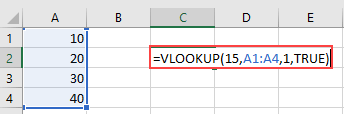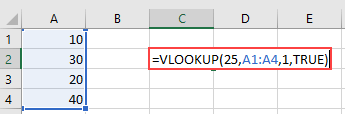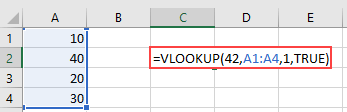Quiz

1. 1
Intermediate

## Suppose cells A1:A4 contain these values: 10, 20, 30, 40. What does the formula =VLOOKUP(15,A1:A4,1,TRUE) return?2. 2
Intermediate

## Suppose cells A1:A4 contain these values: 10, 30, 20, 40. What does the formula =VLOOKUP(25,A1:A4,1,TRUE) return?3. 3
Intermediate

## Suppose cells A1:A4 contain these values: 10, 40, 20, 30. What does the formula =VLOOKUP(42,A1:A4,1,TRUE) return?